Next: Implementation Up: Rohn simplification procedure Previous: Rohn simplification procedure   Contents

Mathematical background

Let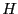be the set of vector with components either -1 or 1 and the scalar matrices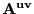,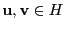,the set of matrices whose elements are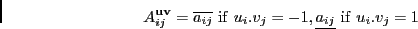The set of matriceshas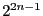elements. If all matrices in the set have a determinant of the same sign, then all the matrices A are regular .

Note than another simplification procedure may be built by using the spectral radius (see section 7.6).

Jean-Pierre Merlet 2012-12-20